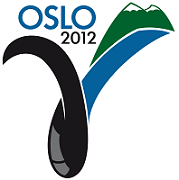Ninth International Geostatistics Congress, Oslo, Norway
June 11 – 15, 2012No Paper Available Session: Posters Abstract No.: P-021 Title: Modeling Nonstationary and Nonlinear Beta Probability Fields Author(s): K.D. Khan, Saudi Aramco (SA)J.A. Vargas-Guzman, Saudi Aramco (SA) Abstract: This study compares direct estimation to kriging under normalizing transform approaches to obtain unbiased parameters for the modeling of local likelihood distributions of proportions or conditional probabilities of a P-field. The motivation comes from a simple exercise that shows how proportions and their probabilities respond to nonlinear and nonstationary Beta models. Perturbed real data and modeled conditional random variables y(x) of an attribute, when projected on the axis of global cumulative probability, F(y) as p(x)=F(y(x)) ? the inverse operation to stochastic simulation ? result in an array of conditional histogram shapes of the [0,1] valued probability random variate p(x). The array of exhaustive histograms resembles the Beta family, f(p(x);,), comprised of a succession of highly flexible shapes on [0,1] ranging from highly skewed, to symmetric, and bimodal for contrasting extreme proportions. The exercise provides empirical evidence that the probability density function (pdf) of conditional probability is Beta-distributed, motivating the investigation of the characteristics and modeling approaches for complex non-linear and non-stationary Beta random fields. P-field simulation introduced the idea of simulating correlated fields of probabilities. The local distributions of probability in P-field simulation were assumed to be uniform. This paper shows that these distributions of conditional probabilities are not uniformly distributed; instead, they follow a wide variety of shapes that can be modeled by the Beta distribution function. Two pathways: direct kriging and simulation, and kriging under a normalizing transformation of the proportions are explored and compared to provide unbiased Beta distribution parameters for simulation. The Beta random field modeling approach is applied to real data, providing a cutting edge analysis and revision of the P-field paradigm for practical and theoretical Geostatistics. Proposals for further development lead to new probability constraint schemes and tools for modeling Beta distributed probability fields of spatially heterogeneous attributes.Produced by X-CD Technologies Inc.